# 市场数学：盈利、亏损、和成本

• 概述
• 计算订单盈亏的公式
• 开盘价和收盘价的点差
• 计算订单盈亏的最精准方法
• 掉期利息精确计算函数
• 实践部分
• 结束语

### 计算订单盈亏的公式

• PrBuy = Lot * TickValue * [ ( PE – PS )/Point ] — 买单盈亏
• PrSell = Lot * TickValue * [ ( PS – PE )/Point ] — 卖单盈亏
• Point — 所选交易品种的最小可能价格变化
• TickValue — 当价格变动 “1” 个点（Point）时，持仓的盈亏值
• PE — 交易收盘价（出价 – Bid）
• PS — 交易开盘价（出价 – Bid）

MQL5 中诸如 Point 和 TickValue 参量值是在预定义变量级别定义的，或者由内置函数 SymbolInfoDouble 返回值的形式提供。 我会在我的文章中定期以这样或那样的方式触及 MQL5 的主题，因为通常只需分析 MQL5 或其某些功能的构建方式，就可以挖掘出许多问题的根底。

• PrBuy = Lot * TickValue * [ ( Bid2 – Ask1 )/Point ] — 买单盈亏
• PrSell = Lot * TickValue * [ ( Bid1 – Ask2 )/Point ] — 卖单盈亏
• Bid1 — 卖出交易开单价
• Bid2 — 买入交易平单价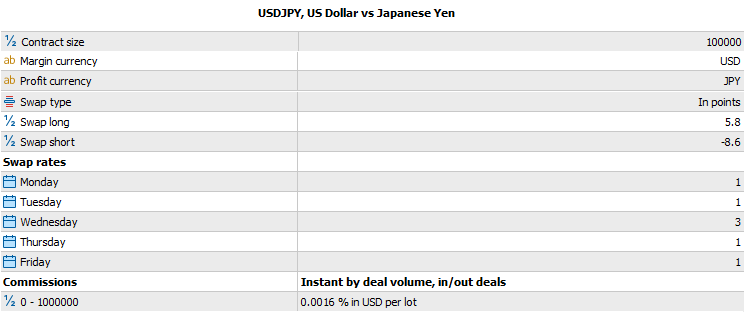1. Comission = Lot * TickValue * ComissionPoints
2. Comission = Lot * ContractSize * BidAlpha * ComissionPercent/100

• ComissionPoints – 佣金以点数为单位
• ComissionPercent – 佣金占合约规模的百分比

1. BidAlpha = 1 (如果基准货币与账户货币相同)
2. BidAlpha = Bid (所选交易品种)
3. BidAlpha = Bid (对应汇率，其中所选交易品种的基准货币与过渡交易品种的基准货币相同，第二种货币与存款货币相同)

1. Swap = Lot * TickValue * SwapPoints * SwapCount(StartTime,EndTime)
2. Swap = Lot * ContractSize * BidAlpha * SwapPercent/100 * SwapCount(StartTime,EndTime)

• SimpleCount = MathFloor( (EndTime -StartTime) / ( 24 * 60 * 60 ) )

• SwapPoints – 以点数为单位的单笔持仓滚延掉期利息
• SwapPercent – 以合约百分比为单位的单笔持仓滚延掉期利息

• PrBuy = Lot * TickValue * [ ( Bid2 – (Bid1+S1*Point) )/Point ] — 买单盈亏
• PrSell = Lot * TickValue * [ ( Bid1 – (Bid2+S2*Point) )/Point ] — 卖单盈亏
• S1 — 开立买单时的点差
• S2 — 开立卖单时的点差

• PrBuy = Lot * TickValue * [ ( Bid2 – Bid1)/Point ] + ( – Lot * TickValue * S1 ) — 买单盈亏
• PrSell = Lot * TickValue * [ ( Bid1 – Bid2)/Point ] + ( – Lot * TickValue * S2 ) — 卖单盈亏

• S1 — 开立任何持仓时的点差
• S2 — 平仓时的点差

• SpreadSell = – Lot * TickValue * S2

### 开盘价和收盘价的点差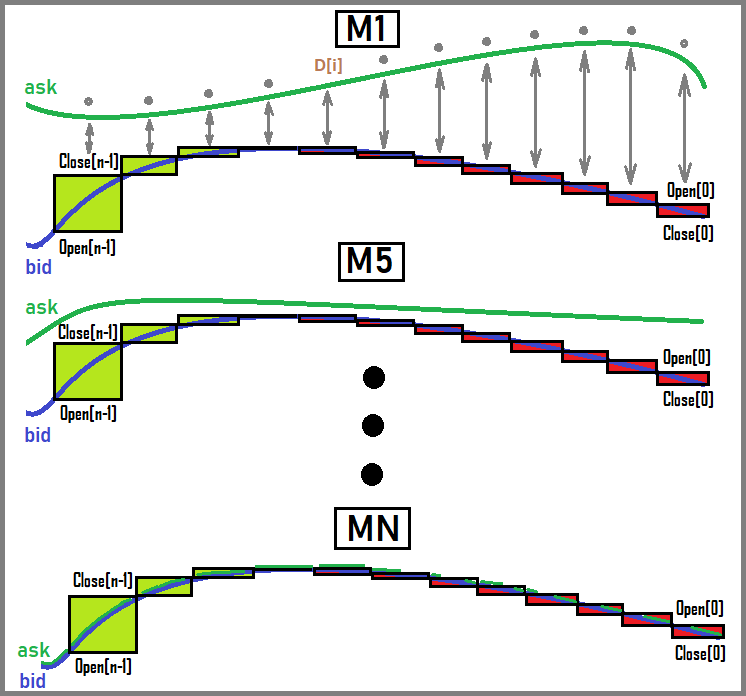• Mid = (Ask + Bid) / 2

• Bid = Mid * 2 – Ask
• Ask = Mid * 2 – Bid

• Bid = Mid * 2 – (Bid + S*Point) = Mid – (S*Point)/2
• Ask = Mid * 2 – (Ask – S*Point) = Mid + (S*Point)/2

• PrBuy = Lot * TickValue * [ ( (Mid2 – (S2*Point)/2) – (Mid1 + (S1*Point)/2) ) )/Point ]
• PrSell = Lot * TickValue * [ ( (Mid1 – (S1*Point)/2) – (Mid2 + (S2*Point)/2) ) )/Point ]

• PrBuy = Lot * TickValue * [ (Mid2 – Mid1)/Point ] – Lot * TickValue * (  S1/2 + S2/2  )
• PrSell = Lot * TickValue * [ (Mid1 – Mid2)/Point ] – Lot * TickValue * (  S1/2 + S2/2  )

• Bid1 = Mid1 – (S1*Point)/2
• Bid2 = Mid2 – (S2*Point)/2
• Ask1 = Mid1 + (S1*Point)/2
• Ask2 = Mid2 + (S2*Point)/2

• Mid1 — 开立任何持仓时市场深度的中间值
• Mid2 — 平仓时市场深度的中间值

• Spread = -Lot * TickValue * (  (S1*Point)/2 + (S2*Point)/2  )

• ProfitIdealBuy = Lot * TickValue * [ (Mid2 – Mid1)/Point ]
• ProfitIdealSell = Lot * TickValue * [ (Mid1 – Mid2)/Point ]

• TotalProfitSell= ProfitIdealSell + (Spread + Comission + Swap)

• Lim[ dP -> 0 ] ( PrBuy(Mid1, Mid1+dP… ) ) = TotalProfitBuy(Mid1, Mid1+dP…)
• Lim[ dP -> 0 ] ( PrSell(Mid1, Mid1+dP… ) ) = TotalProfitSEll(Mid1, Mid1+dP…)
• Mid1+dP = Mid2 — 新价格是取前一个价格，加上趋于零的增量得来的
• TotalProfitSell = TotalProfitSell(P1,P2… ) — 类似

### 计算订单盈亏的最精准方法

• 作者自己并未完全理解，所以他们尽一切办法最大限度地策反您（通常会用反智陈词来达到）
• 故意省略详细信息，从而对您隐瞒不必要的信息。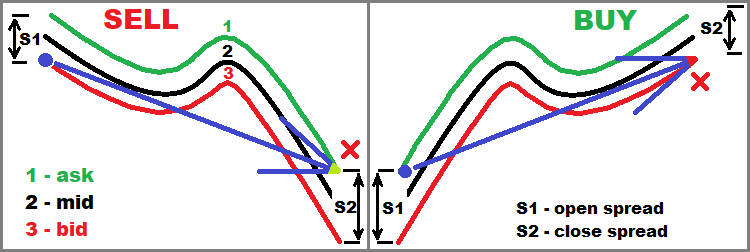• EUR = Lot * ContractSize
• USD = – Ask1 * Lot * ContractSize = – (Bid1 + S1*Point) * Lot * ContractSize

• USD1 = Ask1 * Lot * ContractSize = (Bid1 + S1*Point) * Lot * ContractSize — 这是我们拆借来的
• EUR1 = Lot * ContractSize — 这是我们用拆借来的资金买入的份额，价格取购买时的汇率要价（Ask）

• EUR2 =  Lot * ContractSize
• USD2 = Bid2 * Lot * ContractSize

• Profit_EUR = EUR1 – EUR2 = 0
• Profit_USD = USD2 – USD1 = Bid2 * Lot * ContractSize – (Bid1 + S1*Point) * Lot * ContractSize = Lot * ContractSize * ( Bid2 – Bid1 – S1*Point)

• Profit_USD = Lot * (ContractSize*Point) * [ ( Bid2 – Bid1 – S1*Point) / Point ]

• EUR1 = Lot * ContractSize
• USD1 = – Ask1 * Lot * ContractSize = – (Bid1 + S1*Point) * Lot * ContractSize

• EUR2 = – Lot * ContractSize
• USD2 = Bid1 * Lot * ContractSize

• Profit_EUR = EUR1 + EUR2 = 0
• Profit_USD = USD1 + USD2 = – (Bid1 + S1*Point) * Lot * ContractSize + Bid2 * Lot * ContractSize = Lot * ContractSize * ( Bid2 – Bid1 – S1*Point)

• EUR1 =  – Lot * ContractSize
• USD1 = Bid1 * Lot * ContractSize

• EUR2 = Lot * ContractSize
• USD2 = – (Bid2 + S2*Point) * Lot * ContractSize

• Profit_EUR = EUR1 + EUR2 = 0
• Profit_USD = USD1 + USD2 = Bid1 * Lot * ContractSize – (Bid2 + S2*Point) * Lot * ContractSize = Lot * ContractSize * ( Bid1 – Bid2 – S2*Point)

• TickValue = ContractSize * Point

• TickValueCross = ContractSize * Point * BidAlphaCross

1. 查看我们交易品种中的第二货币（不是基准货币）
2. 寻找包含此货币，以及和我们存款本币的品种
3. 以相应的汇率进行兑换
4. 若有必要，变换品种（镜像）

• BidAlphaCross = PBid

• BuyProfit = BidAlphaCross * Lot * ContractSize * ( Bid2 – Bid1 – S1*Point)
• SellProfit = BidAlphaCross * Lot * ContractSize * ( Bid1 – Bid2 – S2*Point)

• Bid1 = Mid1 – (S1*Point)/2
• Bid2 = Mid2 – (S2*Point)/2

• BuyProfit = BidAlphaCross * Lot * ContractSize * ( Mid2 – (S2*Point)/2 – Mid1 + (S1*Point)/2 – S1*Point)
• SellProfit = BidAlphaCross * Lot * ContractSize * ( Mid1 – (S1*Point)/2 – Mid2 + (S2*Point)/2 – S2*Point)

• BuyProfit = Lot * BidAlphaCross * ContractSize * Point * [ ( Mid2 – Mid1 )/ Point  – ( S1/2 + S2/2 ) ]
• SellProfit = Lot * BidAlphaCross * ContractSize * Point * [ ( Mid1 – Mid2 )/ Point  – ( S1/2 + S2/2 ) ]

• BuyProfit = Lot * TickValueCross * [ ( Mid2 – Mid1 )/ Point ] – Lot * TickValueCross * ( S1/2 + S2/2 )
• SellProfit = Lot * TickValueCross * [ ( Mid1 – Mid2 )/ Point ] – Lot * TickValueCross * ( S1/2 + S2/2 )

### 掉期利息精确计算函数

• Swap = Lot * TickValue * SwapPoints * SwapCount(StartTime,EndTime)
• Swap = Lot * ContractSize * BidAlpha * SwapPercent/100 * SwapCount(StartTime,EndTime)

• TickValue = ContractSize * Point

• TickValueCross = ContractSize * Point * BidAlphaCross

• Swap = Lot * TickValueCross * SwapPoints * SwapCount(StartTime,EndTime)

1. Swap = SUMM(1 … D) { Lot * (SwapPoints * K[i]) * TickValueCross[i] } — 每当 0:00 时日期变更，汇总所有应计掉期利息的点数
2. Swap = SUMM(1 … D) { Lot * ContractSize * BidAlpha[i] * (SwapPercent/100 * K[i]) * } — 按照 %

• K[i] = 1 或 3 — 如果比率为 “3”，这意味着该日应计掉期利息是三倍
• TickValueCross[i] — 掉期利息记贷点的跳价大小的数组
• BidAlpha[i] — 掉期利息收费点的调整费率数组

• TickValueCross[i] = T[i]
• BidAlpha[i] = B[i]
• K[i] = K[i]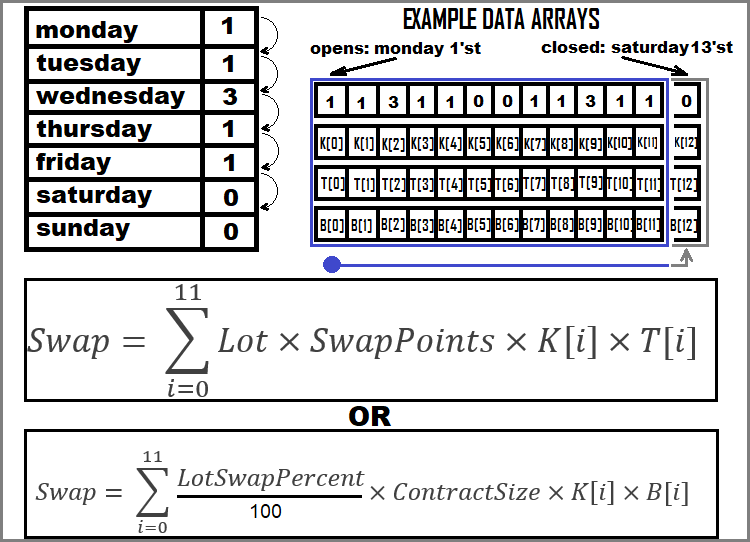### 实践部分

1. Real — 来自历史记录的订单盈利
2. BasicCalculated — 同笔订单，开单时调用 OrderCalcProfit 函数计算的利润
3. CalculatedStart — 在开单时用我们的数学模型计算的利润
4. CalculatedEnd — 在平单时用我们的数学模型计算的利润

1. AverageDeviationCalculatedMQL = Summ(0..n-1) [ 100 * MathAbs(BasicCalculated – Real)/MathAbs(Real) ]  / n : MQL5 代码得出的相对盈利偏差
2. AverageDeviationCalculatedStart = Summ(0.. n-1 ) [  100 * MathAbs(CalculatedStart – Real)/MathAbs(Real) ] / n : 开单时我们自己的代码得出的相对盈利偏差
3. AverageDeviationCalculatedEnd =  Summ(0.. n-1 ) [  100 * MathAbs(CalculatedEnd – Real)/MathAbs(Real) ] / n : 平单时我们自己的代码得出的相对盈利偏差

1. MaxDeviationCalculatedMQL = Max(0.. n-1 ) [ (100 * MathAbs(BasicCalculated – Real)/MathAbs(Real))  ] – MQL5 代码得出的相对盈利偏差
2. MaxDeviationCalculatedStart =  Max(0.. n-1 ) [  (100 * MathAbs(CalculatedStart – Real)/MathAbs(Real)) ]  – 开单时我们自己的代码得出的相对盈利偏差
3. MaxDeviationCalculatedEnd =  Max(0.. n-1 ) [  (100 * MathAbs(CalculatedEnd – Real)/MathAbs(Real)) ]  – 平单时我们自己的代码得出的相对盈利偏差

• Summ(0..n-1) — 所有 “n” 笔订单的全部相对偏差之和
• Max(0..n-1) — 来自所有 “n” 笔订单的最大相对偏差之和

`double CalculateProfitTheoretical(string symbol, double lot,double OpenPrice,double ClosePrice,bool bDirection)     {     //PrBuy = Lot * TickValueCross * [ ( Bid2 - Ask1 )/Point ]     //PrSell = Lot * TickValueCross * [ ( Bid1 - Ask2 )/Point ]     if ( bDirection )        {        return lot * TickValueCross(symbol) * ( (ClosePrice-OpenPrice)/SymbolInfoDouble(symbol,SYMBOL_POINT) );        }     else        {        return lot * TickValueCross(symbol) * ( (OpenPrice-ClosePrice)/SymbolInfoDouble(symbol,SYMBOL_POINT) );        }        }  `

`double TickValueCross(string symbol,int prefixcount=0)     {     if ( SymbolValue(symbol) == SymbolBasic() )        {        return TickValue(symbol);        }     else        {        MqlTick last_tick;        int total=SymbolsTotal(false);//symbols in Market Watch        for(int i=0;i<total;i++) Symbols[i]=SymbolName(i,false);        string crossinstrument=FindCrossInstrument(symbol);        if ( crossinstrument != "" )           {           SymbolInfoTick(crossinstrument,last_tick);           string firstVAL=StringSubstr(crossinstrument,prefixcount,3);           string secondVAL=StringSubstr(crossinstrument,prefixcount+3,3);           if ( secondVAL==SymbolBasic() && firstVAL == SymbolValue(symbol) )              {               return TickValue(symbol) * last_tick.bid;              }           if ( firstVAL==SymbolBasic() && secondVAL == SymbolValue(symbol) )              {              return TickValue(symbol) * 1.0/last_tick.ask;              }                    }        else return TickValue(symbol);          }     return 0.0;        }`

1. 交易品种的盈利货币与我们存款本币相同
2. 所有其它情况（要寻找过渡汇率）

• 存款本币位于转换率的顶部
• 存款本币位于转换率的底部

`string FindCrossInstrument(string symbol,int prefixcount=0)     {     string firstVAL;     string secondVAL;     for(int i=0;i<ArraySize(Symbols);i++)        {        firstVAL=StringSubstr(Symbols[i],prefixcount,3);        secondVAL=StringSubstr(Symbols[i],prefixcount+3,3);        if ( secondVAL==SymbolBasic() && firstVAL == SymbolValue(symbol) )           {           return Symbols[i];           }        if ( firstVAL==SymbolBasic() && secondVAL == SymbolValue(symbol) )           {           return Symbols[i];           }              }     return "";     }`

`string SymbolValue(string symbol,int prefixcount=0)     {     return StringSubstr(symbol,prefixcount+3,3);     }`

`string SymbolBasic()     {     return AccountInfoString(ACCOUNT_CURRENCY);     }`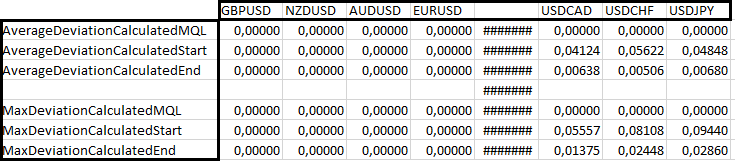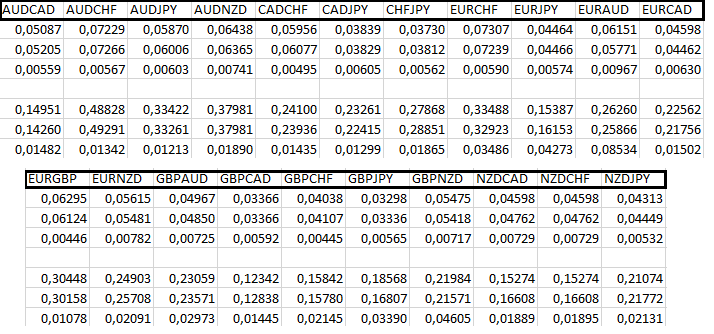### 结束语

ProfiCalculation.mq5 (50.77 KB)

MyFxtops迈投(www.myfxtops.com)-靠谱的外汇跟单社区，免费跟随高手做交易！